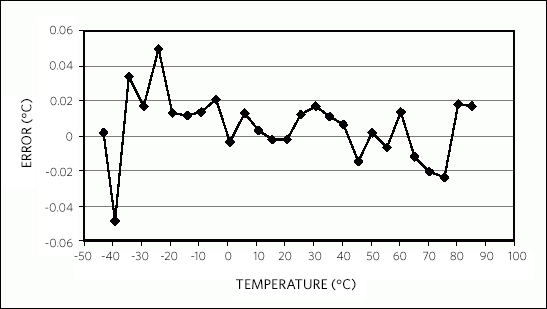# Curve Fitting the Error of a Bandgap-Based Digital Temperature Sensor

### Abstract

A mathematical method is presented in this application note that allows the user to improve the accuracy of bandgap-based digital temperature sensors. This method increases the accuracy by compensating for the offset and curvature of the device error characteristic. This technique is useful for applications that require greater than the ±0.5°C accuracy provided by Maxim Integrated's precision temperature sensor ICs.

### Introduction

Maxim Integrated uses its unique manufacturing capabilities to provide factory-calibrated digital temperature sensors with accuracy as high as ±0.5°C. This level of accuracy is sufficient for many applications; however, some scientific and industrial applications require even greater precision.

This application note presents a technique that can allow the user to improve the accuracy of bandgap-based digital temperature sensors by as much as a factor of 10 by compensating for the offset and curvature of the device error characteristic. This method is successful due to the simple second order error curve and the repeatable nature of the output over temperature for bandgap-based sensors.

Note that the technique presented in this article can be applied to any IC temperature sensor with a bandgap-based thermal circuit. This technique will not work for Maxim's older thermal ICs, which have a dual-oscillator-based thermal measurement circuit.

### Error Compensation

To illustrate the second-order error curve inherent to bandgap-based sensors, a DS1631 IC was characterized over a -35°C to +85°C temperature range in a thermally conductive liquid bath, and its thermal error relative to a NIST traceable platinum resistance temperature detector (RTD) was recorded at 5°C intervals. The results from this sweep are shown in Figure 1 (= DS1631 bath measurements).The error characteristic shown in Figure 1 makes a second-order curve that can be represented by the following equation:

Error = OFFSET + α(TTS - TZERO_SLOPE)2

where TTS is the temperature measured by the temperature sensor (the DS1631 in this case), α is a curvature correction coefficient, TZERO_SLOPE is the temperature at which the error curve has zero slope, and OFFSET is the error at TZERO_SLOPE.

After determining values for α, OFFSET, and TZERO_SLOPE so Equation 1 provides a close fit to the temperature sensor's output error curve, the user can calculate the approximate measurement error at any temperature and then compensate for the error by subtracting the calculated value from the measured temperature. Thus, the compensated temperature is:

TCOMP = TTS - Error = TTS - [OFFSET + α(TTS - TZERO_SLOPE)2]

For the best results with this technique, each temperature sensor should be characterized over the desired temperature range to determine the best fit calculated error curve for the specific device.

### Example

This example uses the characterized DS1631 to illustrate the compensation technique described above. Table 1 lists the values measured by the DS1631 and the error of the measurements relative to the actual ambient temperature. From examining Figure 1, TZERO_SLOPE can be estimated to be 15°C and OFFSET to be approximately -0.23°C, for which α = 1.28 x 10-4 provides a very close fit to the measured error curve (see Figure 1). The compensated temperatures in Table 1 shows the results of plugging the measured temperatures into Equation 2. The error of the compensated temperature relative to the ambient temperature, as shown in Table 1 and Figure 2, is up to 10 times lower than the error of the original measurements.Note that it may take several iterations to arrive at values for TZERO_SLOPE, OFFSET, and α that provide the best fit curve. Once initial estimations for TZERO_SLOPE and OFFSET have been made, α can be calculated with readily available math or spreadsheet software.

 Ambient Temperature* Measured Temperature Measured Error Compensated Temperature Compensated Error -43.1377 -42.9375 0.20015 -43.13603 0.001619 -39.3466 -39.25 0.0966 -39.39572 -0.04912 -34.2368 -34.125 0.11182 -34.20308 0.033737 -29.0969 -29.0625 0.03443 -29.08036 0.016573 -24.1398 -24.125 0.01481 -24.09042 0.049389 -19.2454 -19.3125 -0.06715 -19.23280 0.012547 -14.0779 -14.1875 -0.10964 -14.06626 0.011603 -9.10834 -9.25 -0.14166 -9.09507 0.013266 -4.08784 -4.25 -0.162158 -4.06731 0.020535 0.769446 0.5625 -0.2069459 0.76589 -0.00356 5.831063 5.625 -0.206063 5.84378 0.012717 10.84934 10.625 -0.22434 10.85256 0.003216 15.79473 15.5625 -0.23223 15.79246 -0.00227 20.79082 20.5625 -0.22832 20.78855 -0.00227 25.70361 25.5 -0.20361 25.71593 0.012315 30.74484 30.5625 -0.18234 30.76158 0.016741 35.60317 35.4375 -0.16567 35.61418 0.011006 40.57861 40.4375 -0.14111 40.58489 0.006284 45.68797 45.5625 -0.12547 45.67325 -0.01472 50.56738 50.5 -0.06738 50.56911 0.001733 55.58933 55.5625 -0.02683 55.58245 -0.00688 60.51409 60.5625 0.04841 60.52748 0.01339 65.35461 65.4375 0.08289 65.34273 -0.01188 70.54241 70.6875 0.14509 70.52161 -0.0208 75.40949 75.625 0.21551 75.38579 -0.0237 80.41012 80.75 0.33988 80.42811 0.017987 85.26303 85.6875 0.42447 85.27961 0.016576 *As measured by a NIST traceable platinum RTD.